# DC/DC converter datasheets: current limit - part one

The current-limit specification for a DC/DC converter can sometimes cause confusion for designers not familiar with this type of regulator. In this two-part series, I hope that I can help clear away some of the confusion.

First, the current-limit specification in a DC/DC converter data sheet does not mean the same thing as it does for a low-dropout regulator (LDO). For an LDO, the current-limit value is the maximum current that the device will provide to the output when the regulator is in an overload or short-circuit condition. For a step-down converter, the data sheet will specify the limit on either the peak or valley of the inductor current. However, it is the average inductor current that represents the output current of a buck converter. Equations 1 and 2 translate the inductor current limits into the maximum output current: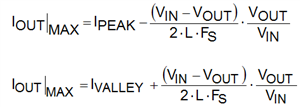As an example, let's take the LMR16030 converting a 24V input to a 5V output. As shown in Figure 1, the minimum peak-current limit given in the data sheet is 3.8A.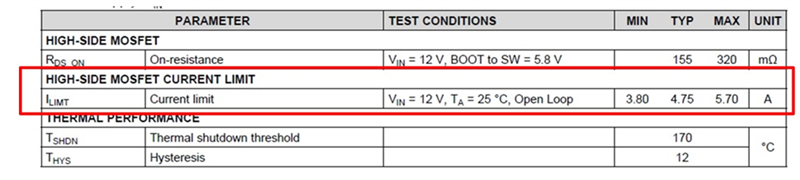Figure 1: Current Limit Specification for LMR16030

Using Equation 1, with the inductor chosen in the data sheet example, you have: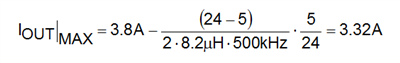Note that this is a “3A” regulator, yet you can get somewhat more than 3A of load current under these conditions.

Take the LM43601 as another example. As Figure 2 shows, the data sheet specifies a typical peak-current limit of 2.45A and a typical valley-current limit of 1.1A.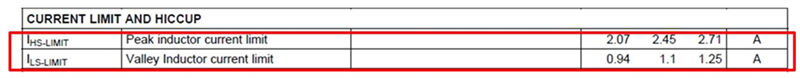Figure 2: Current Limit Specification for LM43601

Using Equations 1 and 2, you have: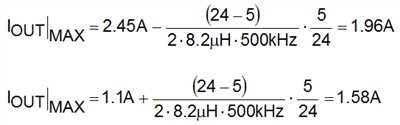You would take the lower value of 1.58A as your maximum output current for this device under these conditions.

The previous calculation represents the maximum output current before the converter becomes overloaded and the output falls out of regulation. For the more severe condition of a short circuit on the output, most regulators will reduce the maximum output current even more. This "fold-back" mode helps prevent overheating of the converter and damage to the power-stage components.

In part 2, I will consider the step-up or boost converter. In the meantime, consider one of TI’s DC/DC converters for your next design.